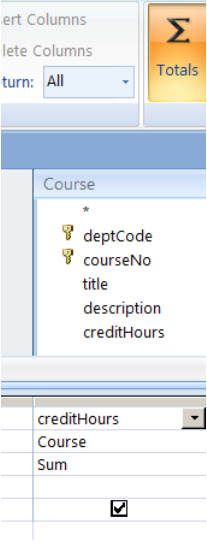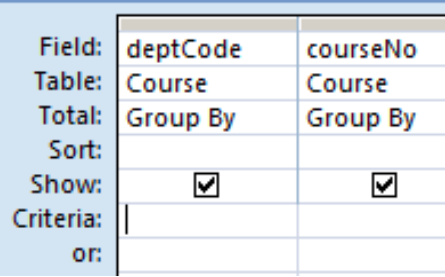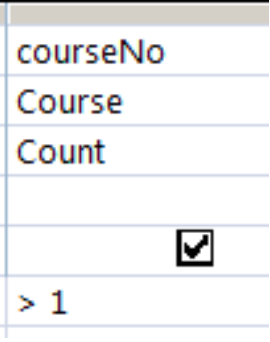# 6.4: Totals Query

$$\newcommand{\vecs}{\overset { \rightharpoonup} {\mathbf{#1}} }$$ $$\newcommand{\vecd}{\overset{-\!-\!\rightharpoonup}{\vphantom{a}\smash {#1}}}$$$$\newcommand{\id}{\mathrm{id}}$$ $$\newcommand{\Span}{\mathrm{span}}$$ $$\newcommand{\kernel}{\mathrm{null}\,}$$ $$\newcommand{\range}{\mathrm{range}\,}$$ $$\newcommand{\RealPart}{\mathrm{Re}}$$ $$\newcommand{\ImaginaryPart}{\mathrm{Im}}$$ $$\newcommand{\Argument}{\mathrm{Arg}}$$ $$\newcommand{\norm}{\| #1 \|}$$ $$\newcommand{\inner}{\langle #1, #2 \rangle}$$ $$\newcommand{\Span}{\mathrm{span}}$$ $$\newcommand{\id}{\mathrm{id}}$$ $$\newcommand{\Span}{\mathrm{span}}$$ $$\newcommand{\kernel}{\mathrm{null}\,}$$ $$\newcommand{\range}{\mathrm{range}\,}$$ $$\newcommand{\RealPart}{\mathrm{Re}}$$ $$\newcommand{\ImaginaryPart}{\mathrm{Im}}$$ $$\newcommand{\Argument}{\mathrm{Arg}}$$ $$\newcommand{\norm}{\| #1 \|}$$ $$\newcommand{\inner}{\langle #1, #2 \rangle}$$ $$\newcommand{\Span}{\mathrm{span}}$$$$\newcommand{\AA}{\unicode[.8,0]{x212B}}$$

A Totals query allows you to summarize information in the database. When you summarize data from one or more tables then either:

• you are producing summary data for the whole table, or
• you are producing summary data for specific groups.

For instance, you may want to know

• how many courses there are
• the average of the credit hours
• the number of courses in each department

To create a Totals query you begin by creating a simple query that retrieves all the attributes that will be needed in the summarization, and then click the Totals icon found in the upper-right hand corner of the MS Access window:Figure $$\PageIndex{1}$$: Totals icon.

When you click the Totals icon the Grid changes to include a Total line. For each field in the grid you must choose from the drop down:Figure $$\PageIndex{2}$$: Choices for the Total line.

For each field in the grid you choose one of:

• “Group By”: if the field is used for grouping
• An aggregate function: if the field is to be summarized using that function. We will consider the standard set including sum, average, minimum, maximum, count.
• “Where”: if the field has criteria to be used for selecting rows. Only rows satisfying the criteria contribute to the grouping and display of results.

Example

The simplest type of totalling query displays an aggregate over an entire set of rows. For example, to sum the credit hours over all courses in the University database one can use:Figure $$\PageIndex{3}$$: Determining the total for one field over all rows.

This query summarizes the entire table. The result of this query is one line displaying a sum.

Example

Typically the use of the Totalling feature is more complicated. Consider the University database and that we need to obtain a count of the number of courses offered by each department. We begin with a query that lists the department code and any other field of the Course table (courseNo is a good choice because it can never be null – nulls are passed over when the counting of field values is performed). The query below lists the fields we need:Figure $$\PageIndex{4}$$: Step 1: the fields needed.

In the upper right-hand corner of Design View for queries you must click the Totals icon. When you click the Totals icon a new line (Total line) is added to the grid:Figure $$\PageIndex{5}$$: Step 2: Total line is added to the grid.

By default, MS Access sets each field up for grouping. To count the number of courses in each department you must click in the Total area for courseNo and change from Group By to Count:Figure $$\PageIndex{6}$$: Step 3: Choose the appropriate aggregate for the group.

Now you have a query that will show the value of each department code along with a count of the number of courses for the department. This query produces one row per department.

Note the choices for aggregate functions – the first 5 are part of the SQL standard: SUM, AVG, MIN, MAX, COUNT and perform a sum, average, minimum, maximum or count over the values found within a group. When a Totalling query is executed, the following actions are performed by MS Access:

1. Rows are retrieved from the underlying table(s): Recall that when Where is specified in the Total line then there is criteria that must evaluate to true for a row to be part of this result).
2. The retrieved rows are organized into groups where the rows forming a group have the same value for the grouping field(s).
3. For each group aggregates are evaluated.
4. A group can be eliminated from the results: If there is a criteria specified for a group then if the criteria evaluates to false the group is excluded.

## Exercises

Write queries for:

Exercise $$\PageIndex{1}$$

Consider the last example where the number of courses per department is listed. The sample database is small and so many departments have just 1 course. Modify the query to list results only for departments where there is more than 1 course. For this you must include a criteria >1 for the field where COUNT is specified:Exercise $$\PageIndex{2}$$

Consider the University database:

1. For each department list the department code and the largest value for credit hours.
2. For each department list the department code, department name, and the number of courses.

Exercise $$\PageIndex{3}$$

Consider the Library database:

1. List the number of books that have SQL in the title.
2. List the number of members by gender.
3. What is the total for fines?

Exercise $$\PageIndex{4}$$

Consider the Company database

1. List the number of employees in each department.
2. List departments that have more than 25 employees.
3. For each employee who is a supervisor, list the supervisor name and the number of employees they supervise.
4. Suppose the Employee table has a salary field holding an employee’s salary. What is the average salary?